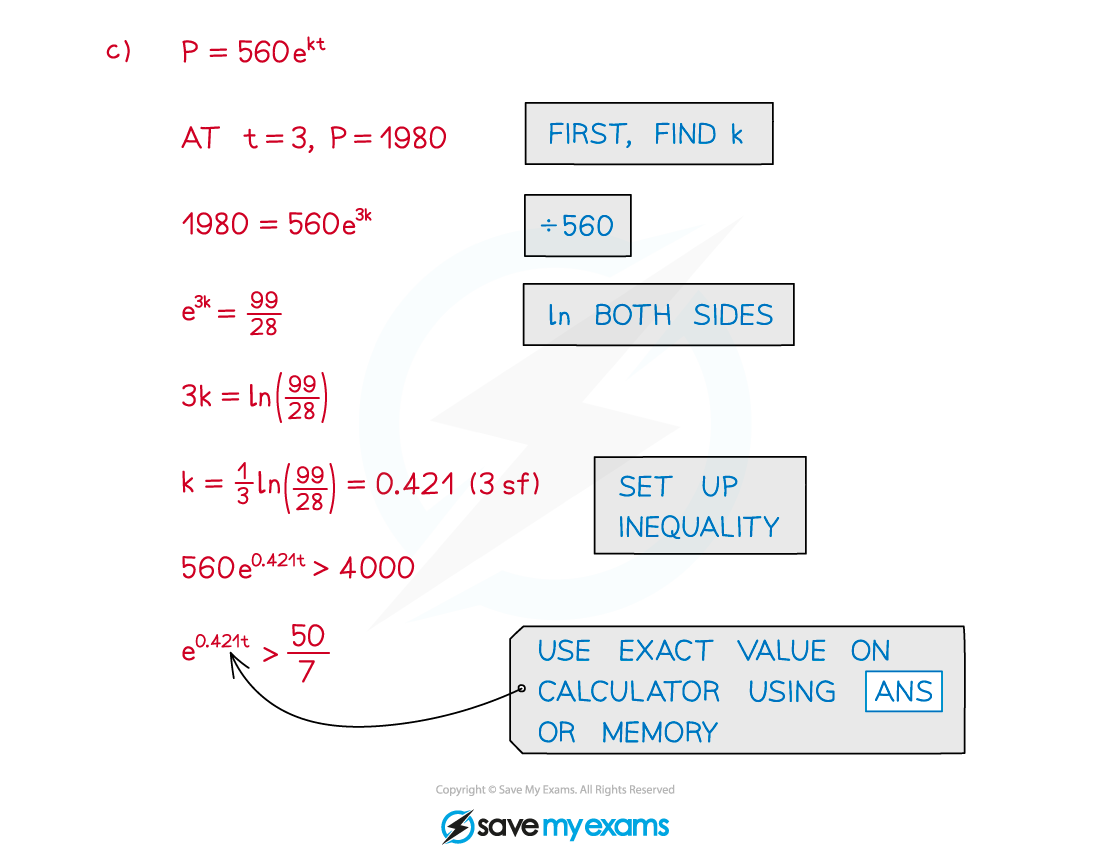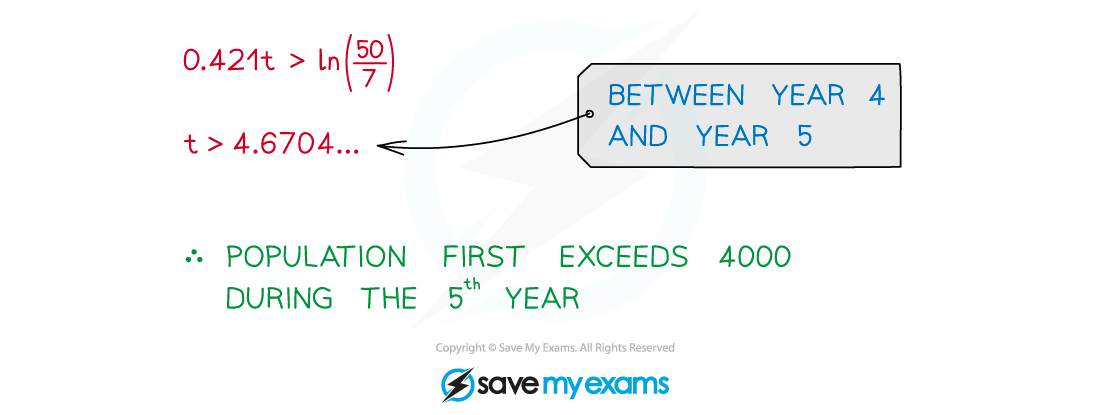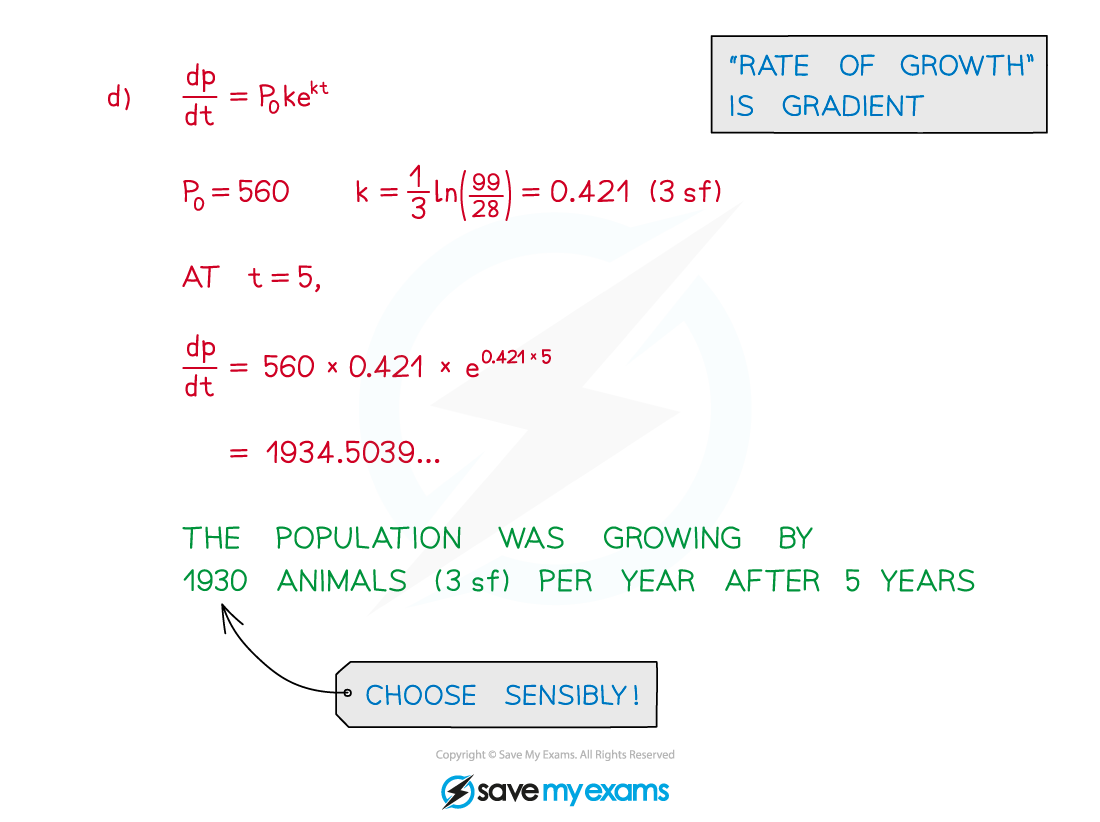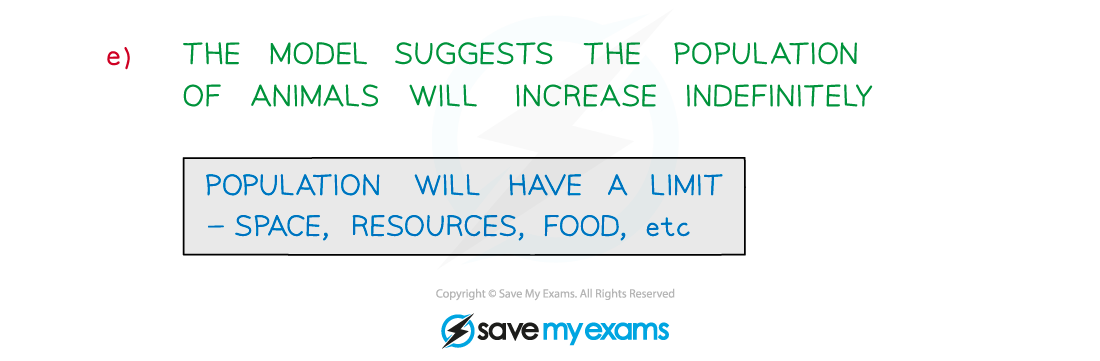# AQA A Level Maths: Pure复习笔记6.3.2 Using Exps & Logs in Modelling

### Using Exps & Logs in Modelling

#### What is exponential modelling?

• Exponential modelling is where real-life situations are modelled using exponential growth and decay
• Exponential growth is typically used for …
• … population (animals and humans) increase
• … the value of an investment under compound interest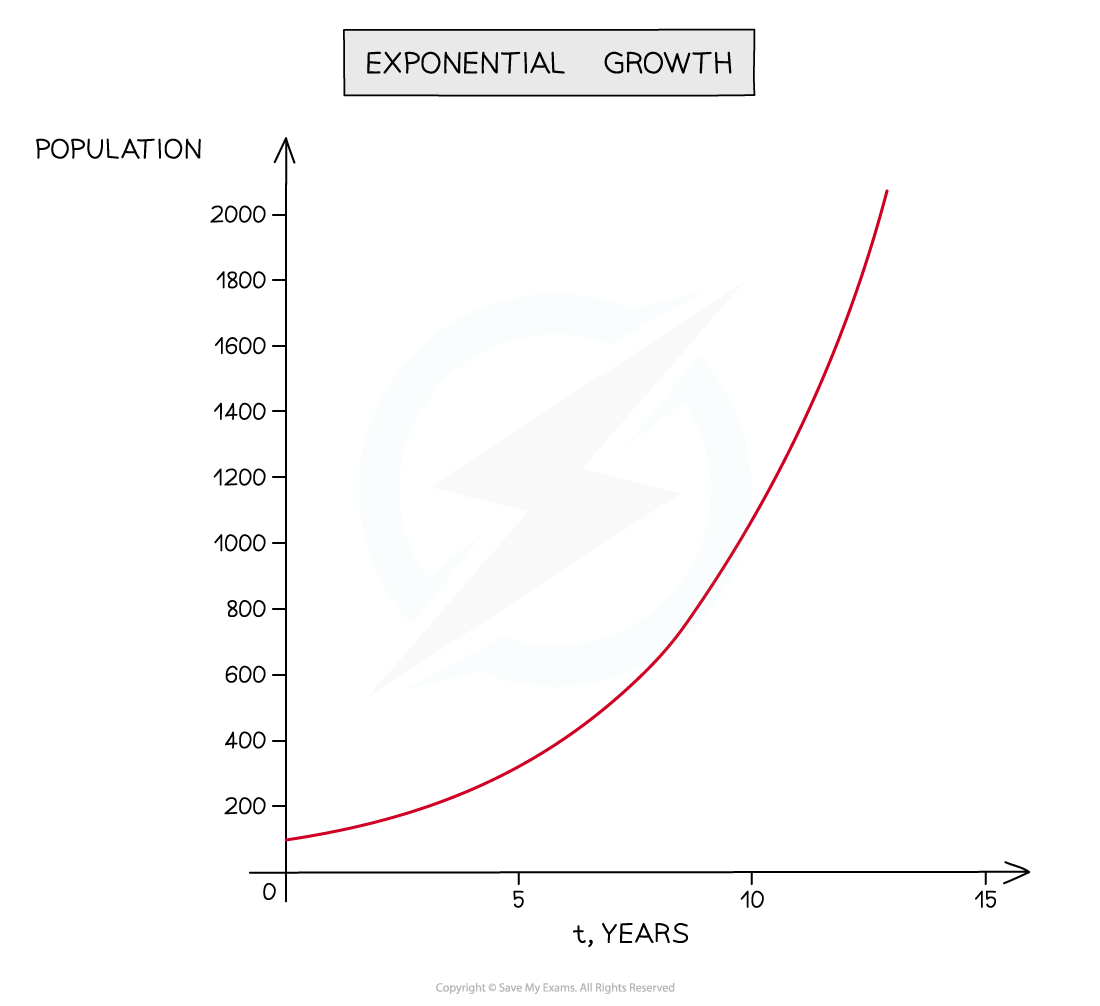•  Exponential decay is typically used for …
• … levels of radioactivity
• … quantity of a drug in a person’s bloodstream
• … depreciation (eg value of a standard car)• It is important to consider if a model is only relevant for a certain period of time
• Can a population increase forever?
• Will a very old car still be worth a small amount of money?
• For example: Consider a substance that initially contains 2000 radioactive atoms where the number of radioactive atoms, N, at time, t hours, is modelled by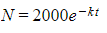• y is N
• A = 2000 – the initial number of radioactive atoms
• k > 0 so the negative indicates this must be exponential decay
• t is used (rather than x) as the situation is time-dependent

#### How do I solve modelling problems?

• Recognise the form of an exponential model
• Growth y = Aekt
• Decay y = Ae-kt
• A (constant) is the starting or initial value
• When t = 0,ekt = e0 = 1

so   y = A

• k (constant) determines the rate of growth/decay
• Many modelling problems are about rates of change –
• If y = Aektthen     dy/dx = Akekt
• If y = Ae-ktthen     dy/dx = -Ake-kt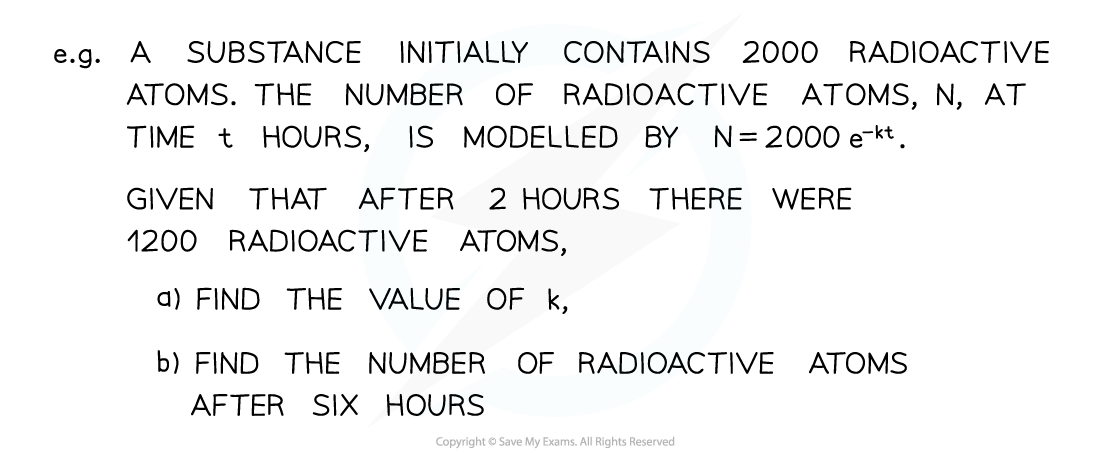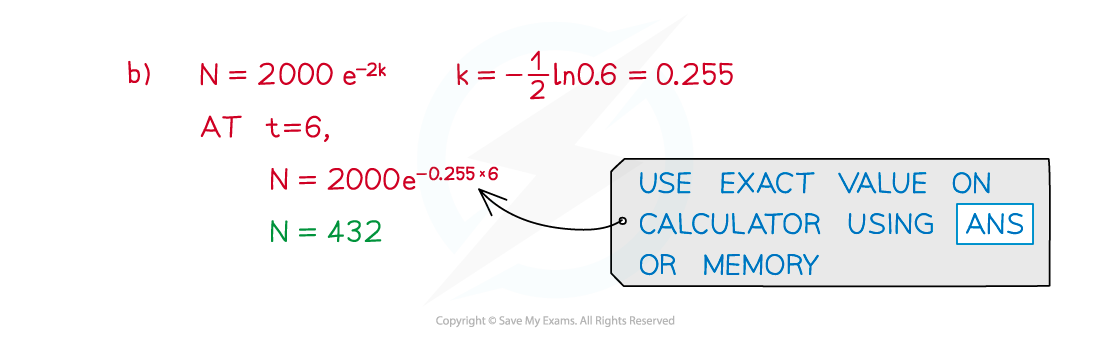#### Worked Example